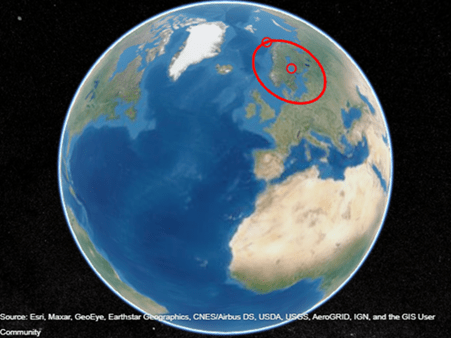# Generate Small Circles

A small circle is the intersection of a plane with the surface of a sphere, such as a parallel of latitude on a spherical representation of the Earth. You can generate a small circle from the center point and a point on the perimeter by using the `scircle2` function. You can generate a small circle from the center point and the arc length of the radius by using the `scircle1` function.

For this example, create a small circle that surrounds Norway, Sweden, and Finland by using the `scircle2` function and then create an identical small circle by using the `scircle1` function.

First, specify the coordinates of a center point and a point along the perimeter. Find the coordinates of 100 points that make up the small circle by using the `scircle2` function.

```centerLat = 62; centerLon = 20; perimeterLat = 72; perimeterLon = 19; [lat2,lon2] = scircle2(centerLat,centerLon, ... perimeterLat,perimeterLon);```

Calculate the arc length in degrees between the center point and the point along the perimeter. Find the coordinates of the same small circle by using the `scircle1` function.

```[arclen,~] = distance(centerLat,centerLon, ... perimeterLat,perimeterLon); [lat1,lon1] = scircle1(centerLat,centerLon,arclen);```

Verify that the small circles are identical, within a tolerance.

```dLat = abs(lat1-lat2); dLon = abs(lon1-lon2); all(dLat < 1e-12)```
```ans = logical 1 ```
`all(dLon < 1e-12)`
```ans = logical 1 ```

Display the center point, perimeter point, and small circle on the surface of a geographic globe. Change the view by using the `campos` function.

```uif = uifigure; g = geoglobe(uif,'Terrain','none'); hold(g,'on') geoplot3(g,centerLat,centerLon,0,'ro','LineWidth',2) geoplot3(g,perimeterLat,perimeterLon,0,'ro','LineWidth',2) geoplot3(g,lat1,lon1,0,'r','LineWidth',2) campos(g,40,-18,10000000)```